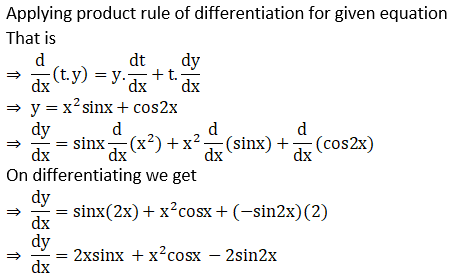# NCERT Exemplar Solutions for Class 11 Maths Chapter 13 Limits and Derivatives

NCERT Exemplar Solutions for Class 11 Maths Chapter 13 Limits and Derivatives provide comprehensive solutions for all the questions in the NCERT Exemplar textbook. These solutions will increase the level of confidence in students, as the concepts are clearly explained and structured, which will help them excel in the annual examinations. The solutions are prepared and reviewed by the subject-matter experts at BYJU’S, and they are revised according to the latest CBSE syllabus and NCERT guidelines. These books are widely used by students who wish to excel in the final exams as they provide a vast number of questions to solve. With the help of NCERT Exemplar Solutions, every student will be capable of solving complex problems in each exercise.

NCERT Exemplar Solutions for Class 11 Maths Chapter 13, Limits and Derivatives, are the best study material for students to excel in exams. This chapter explains the limits of a function. We have provided answers to NCERT Exemplar Solutions in PDF, which can be downloaded easily from the below-provided link. These solutions are helpful for the students to clarify their doubts and build a strong foundation for every concept. Some of the essential topics of the chapter are listed below.

• Limits of a function
• Some properties of limits
• Limits of polynomials and rational functions
• Limits of trigonometric functions
• Derivatives
• Algebra of derivatives of functions

## Download the PDF of NCERT Exemplar Solutions for Class 11 Maths Chapter 13 Limits and Derivatives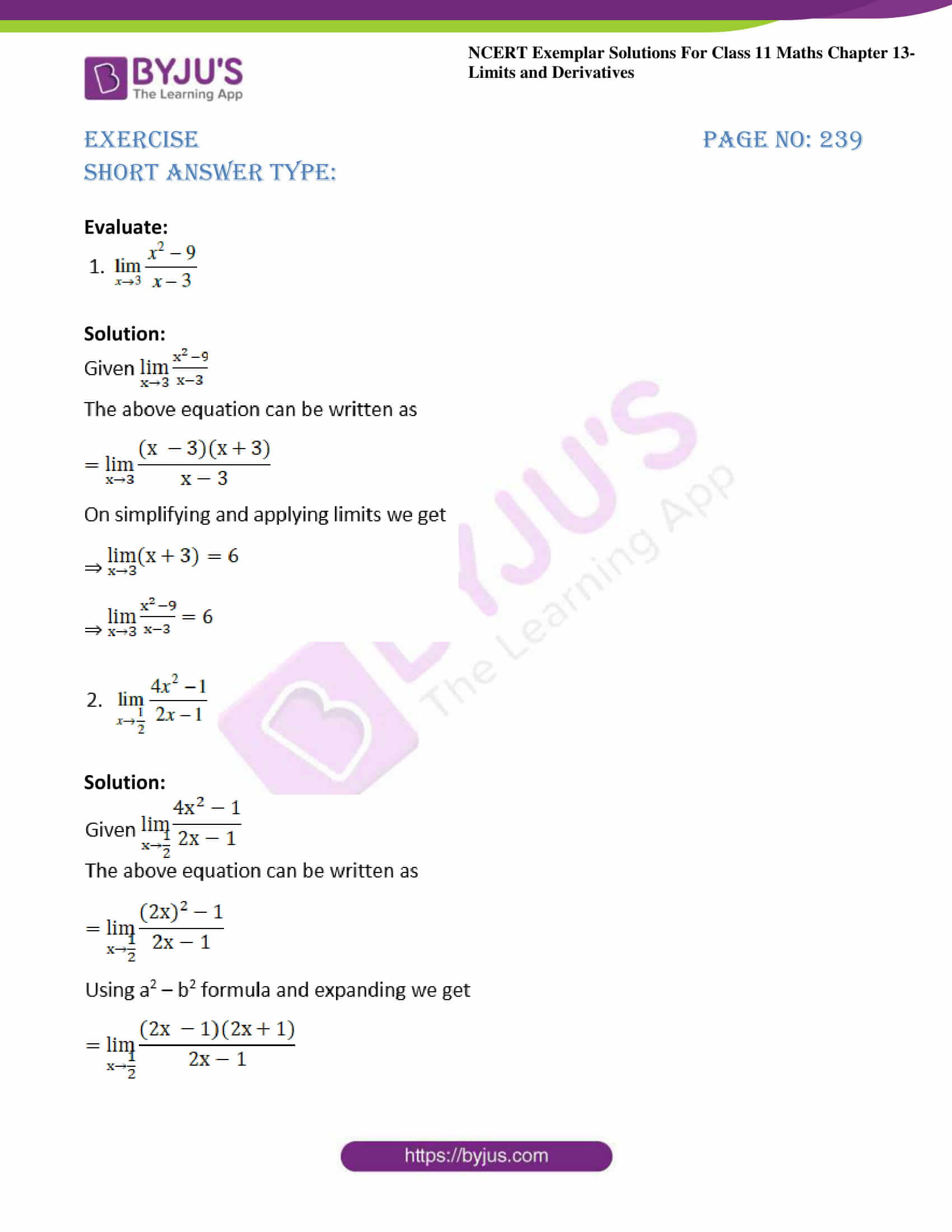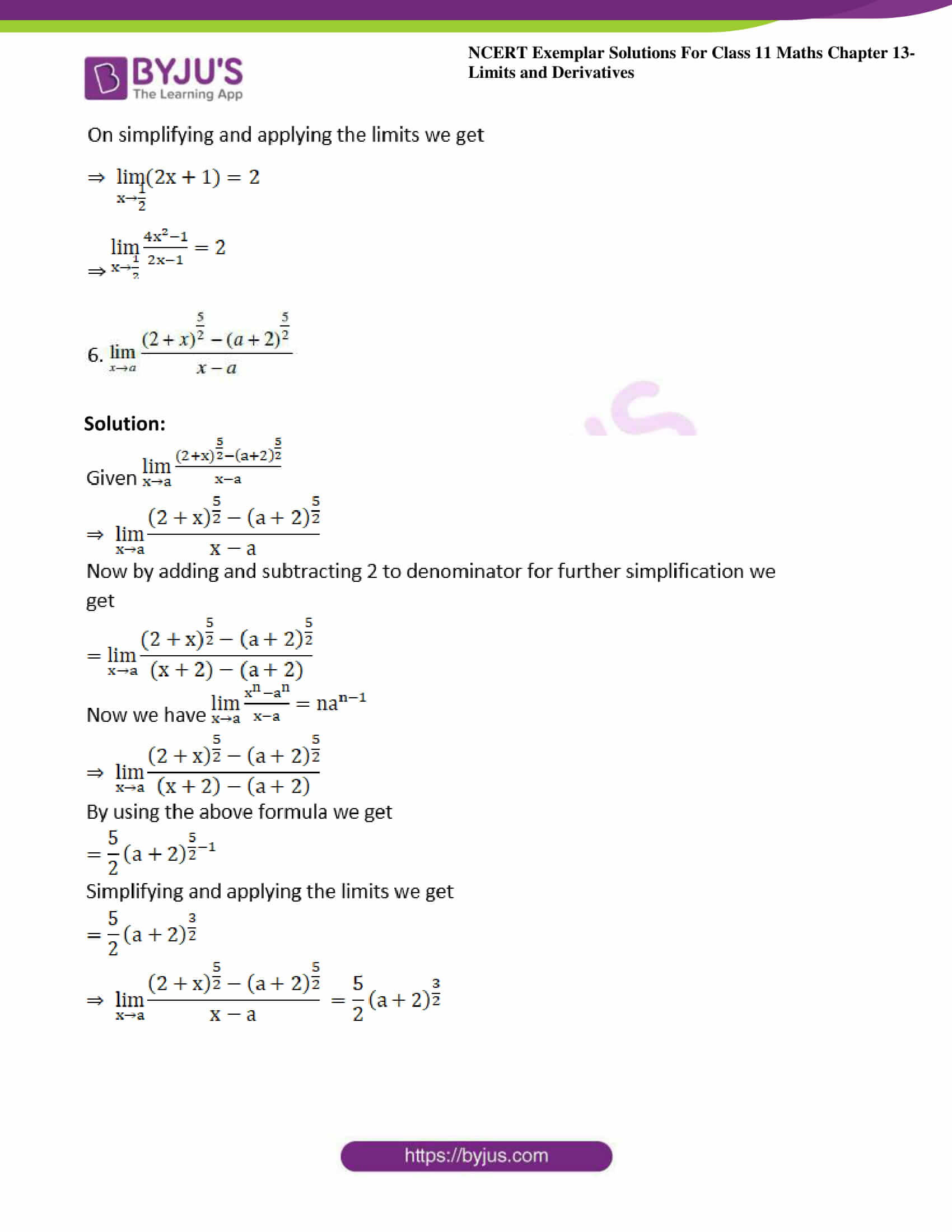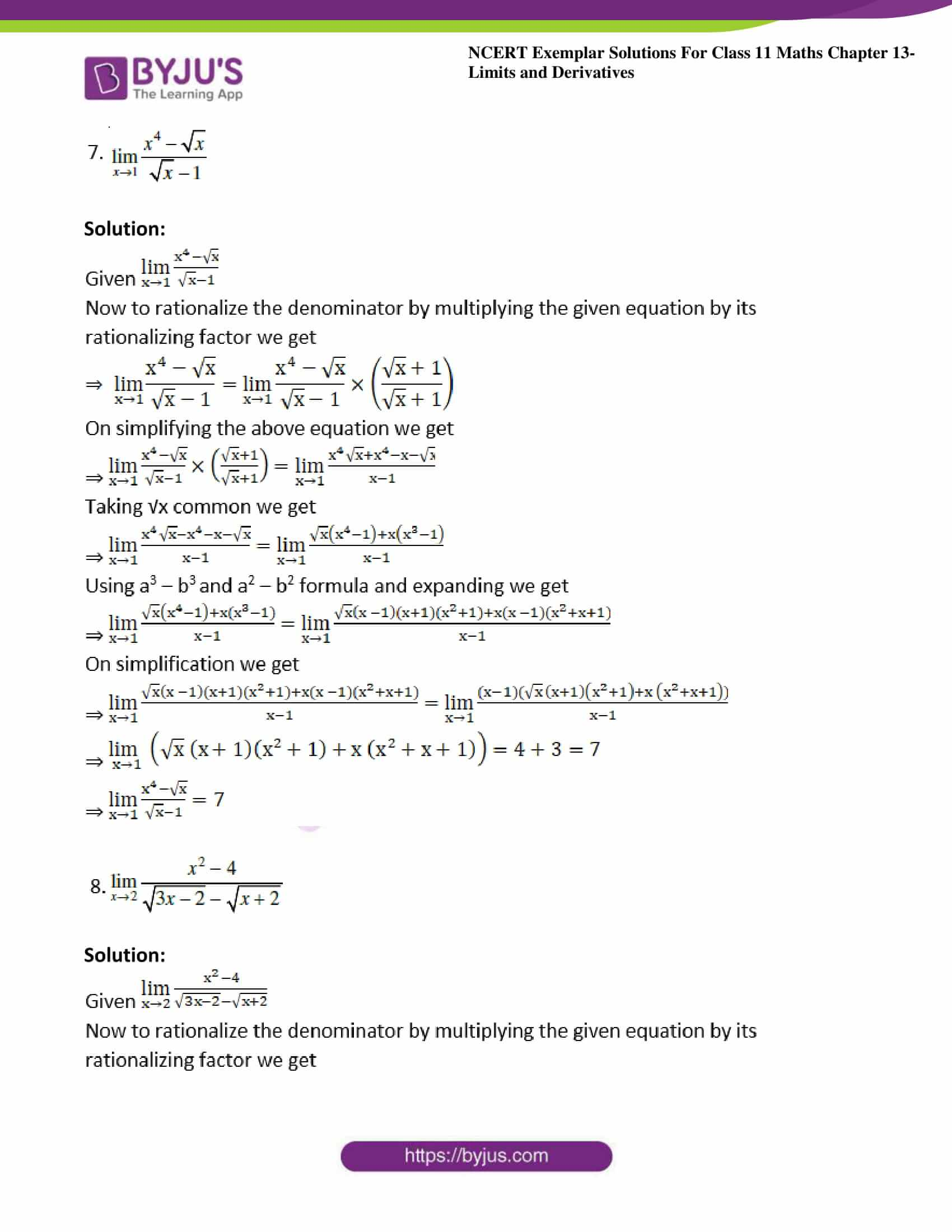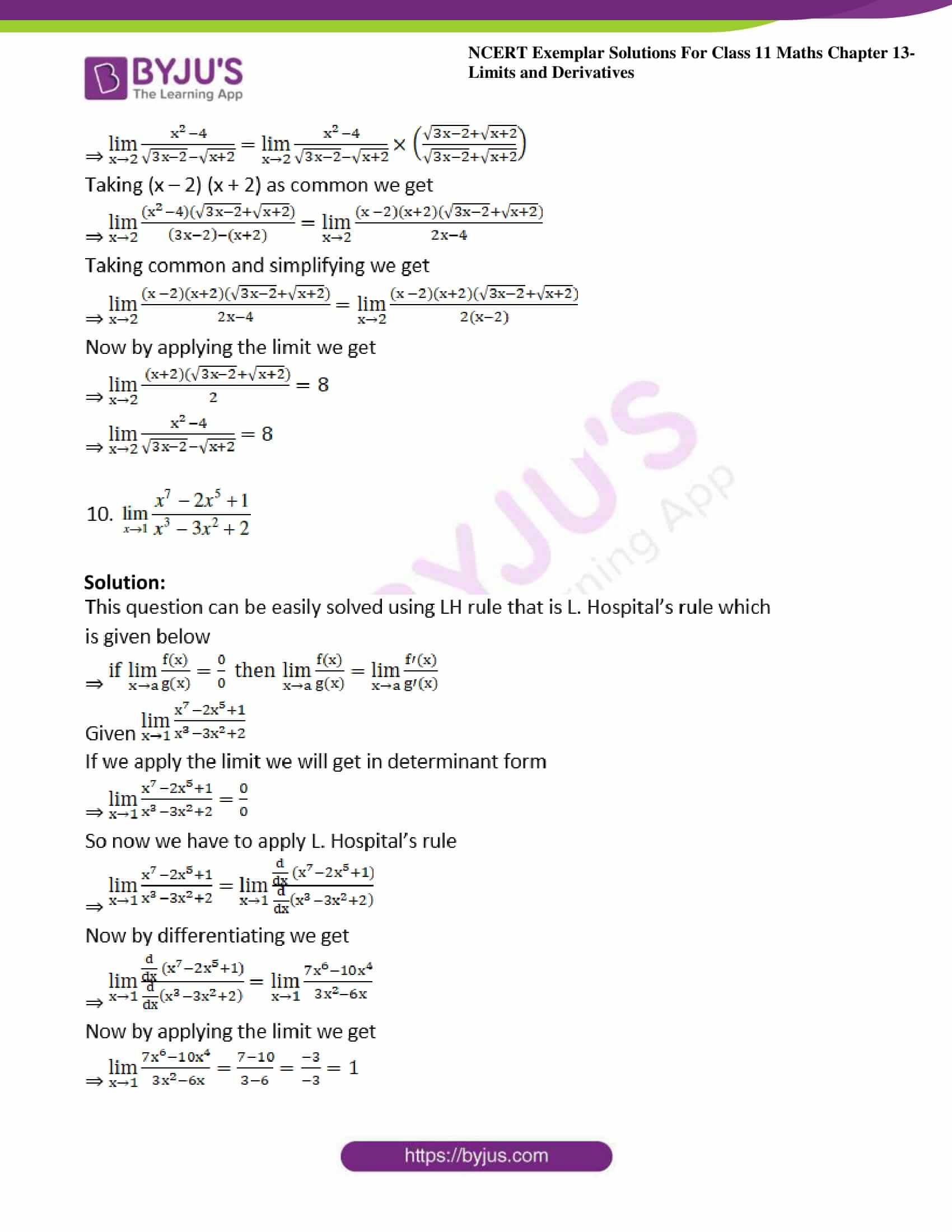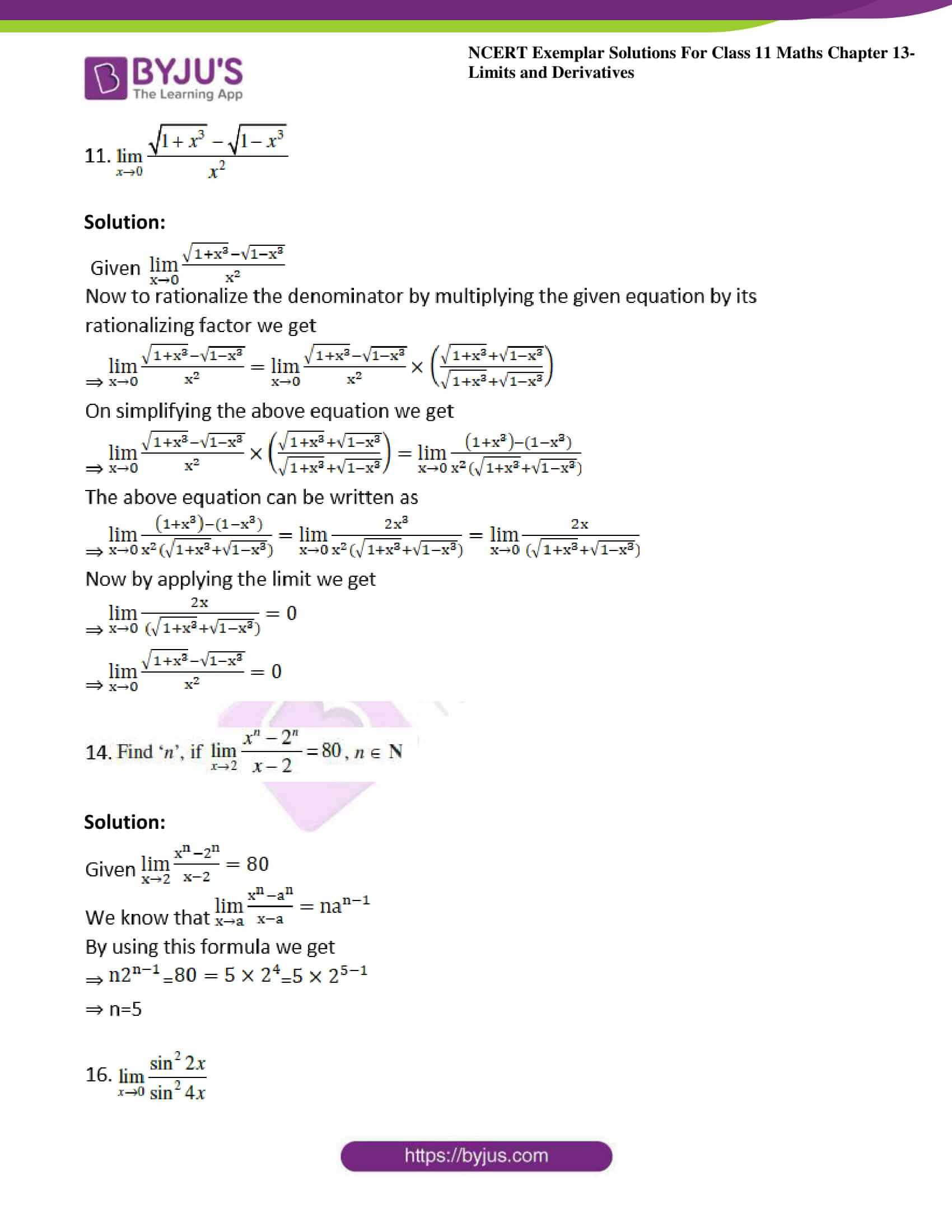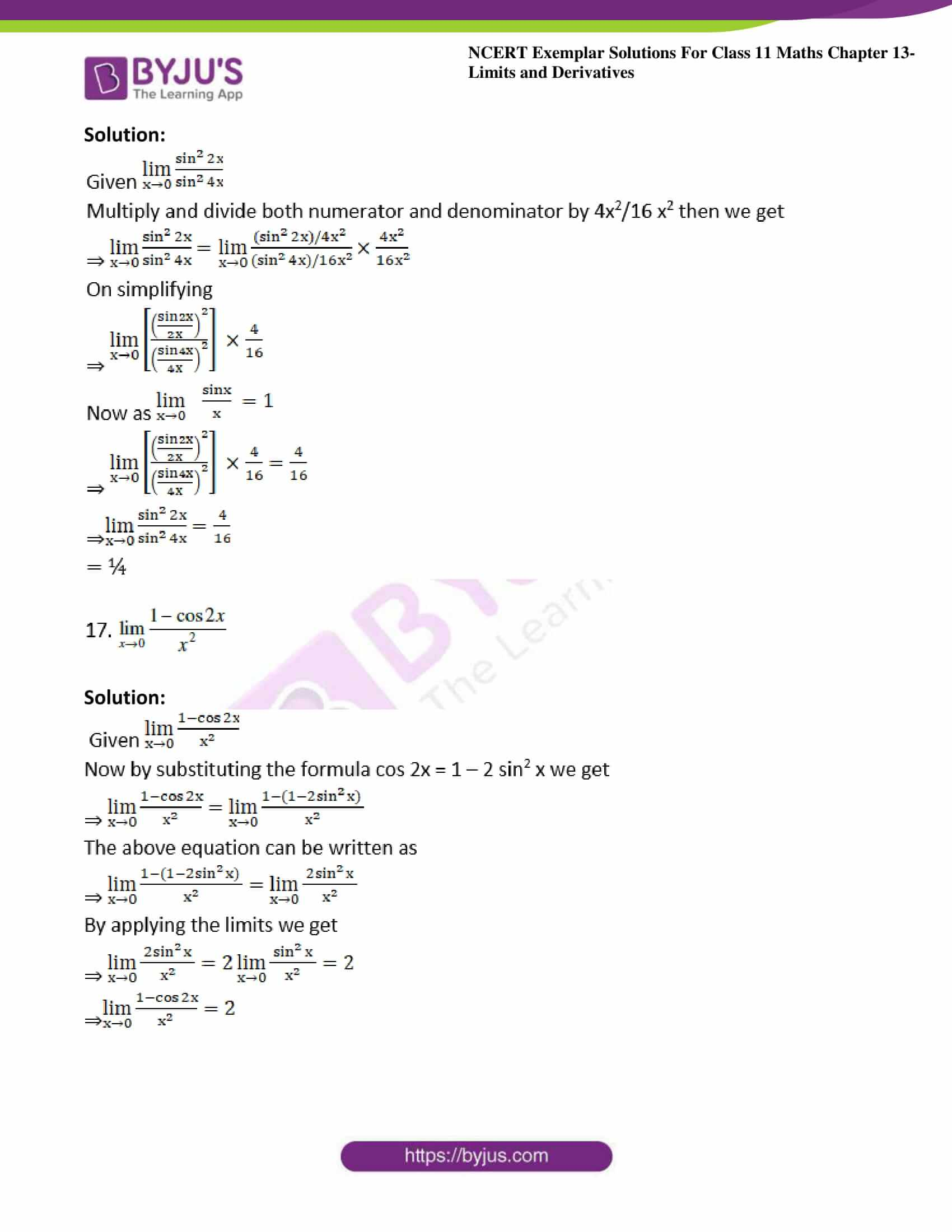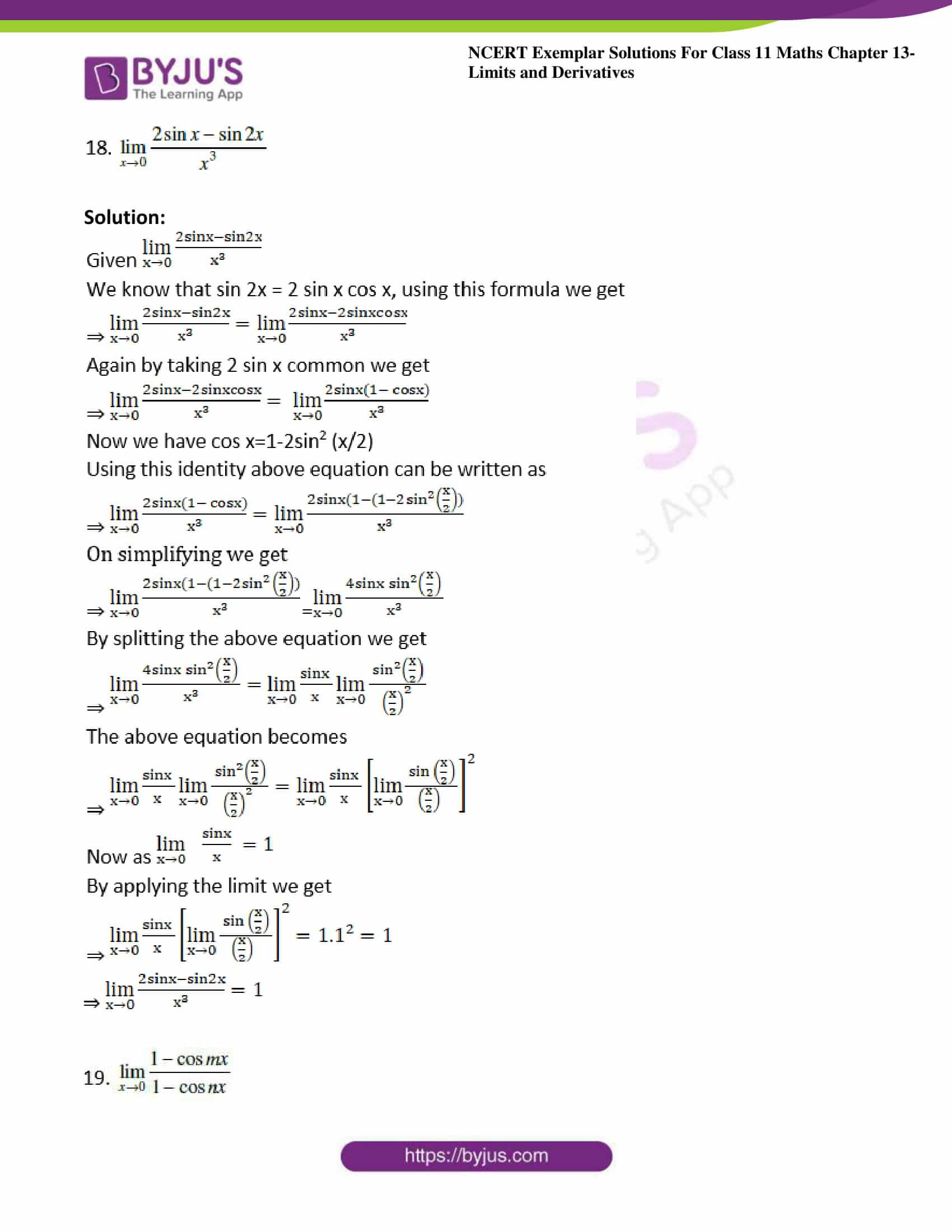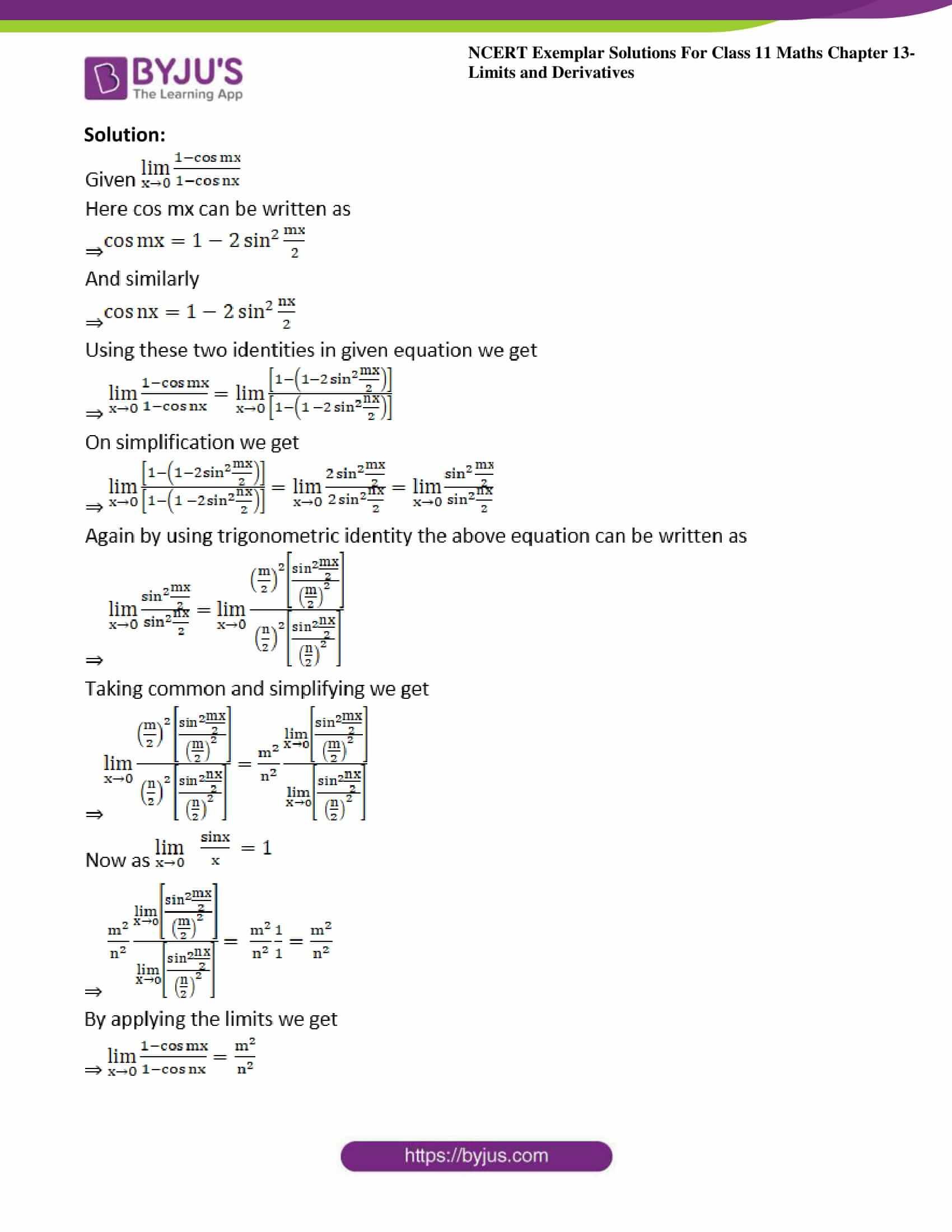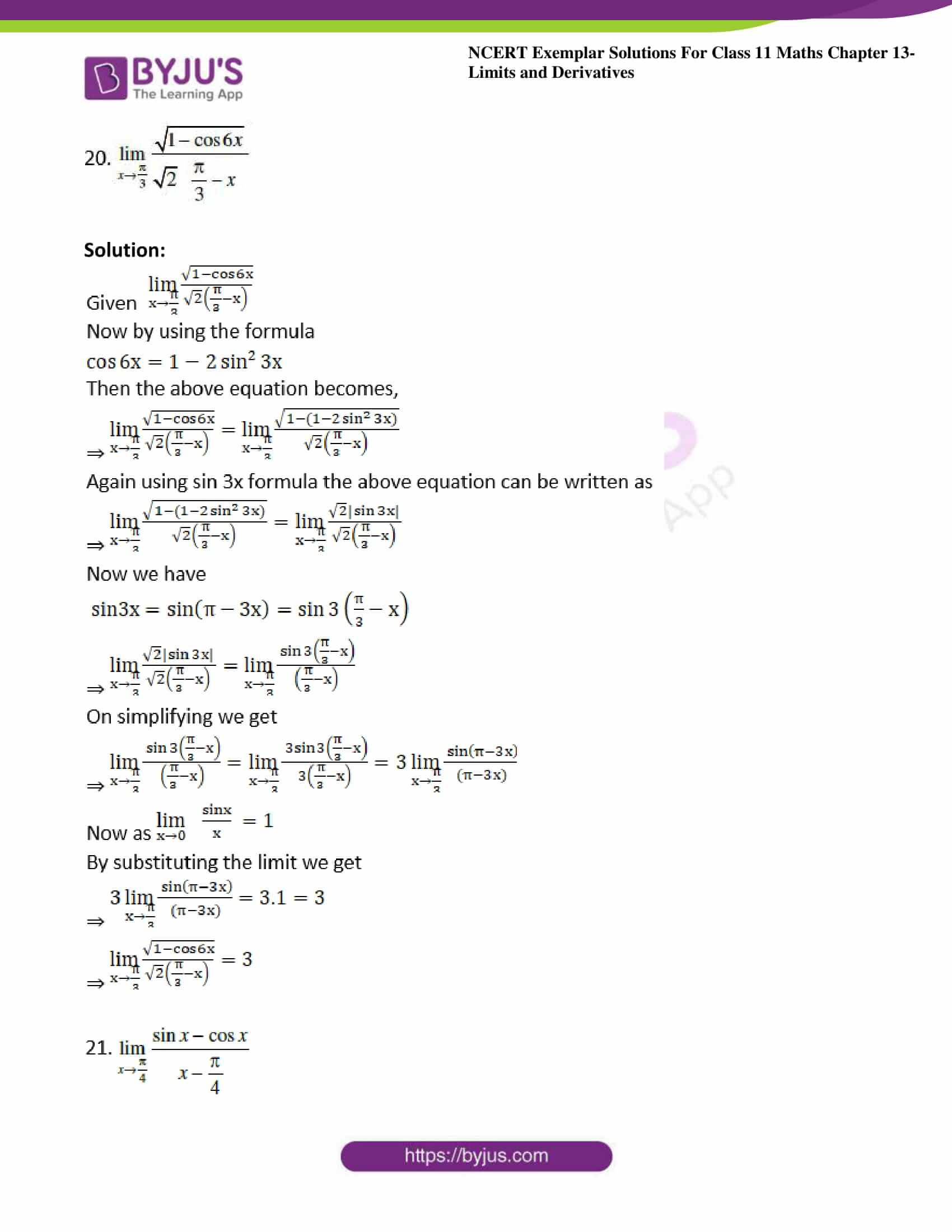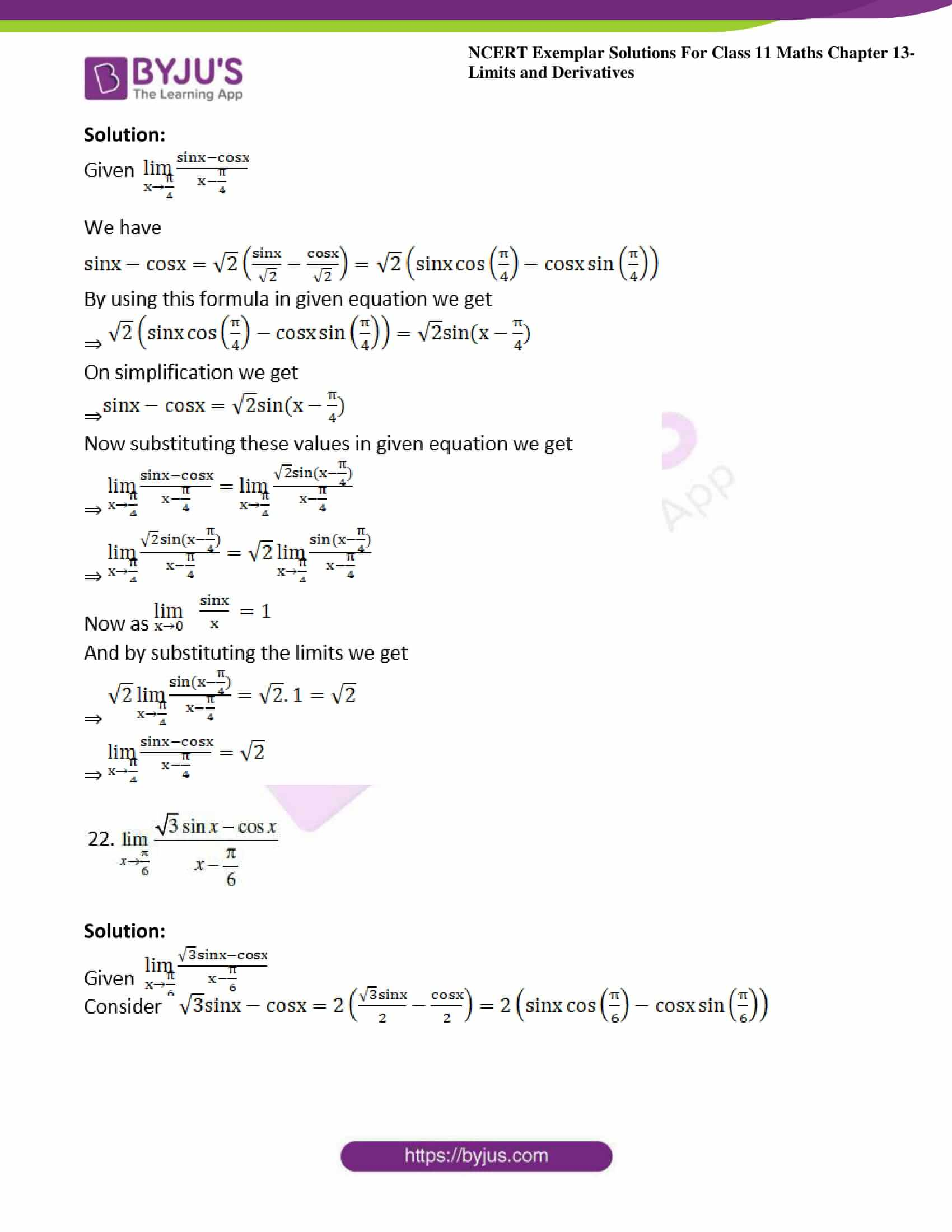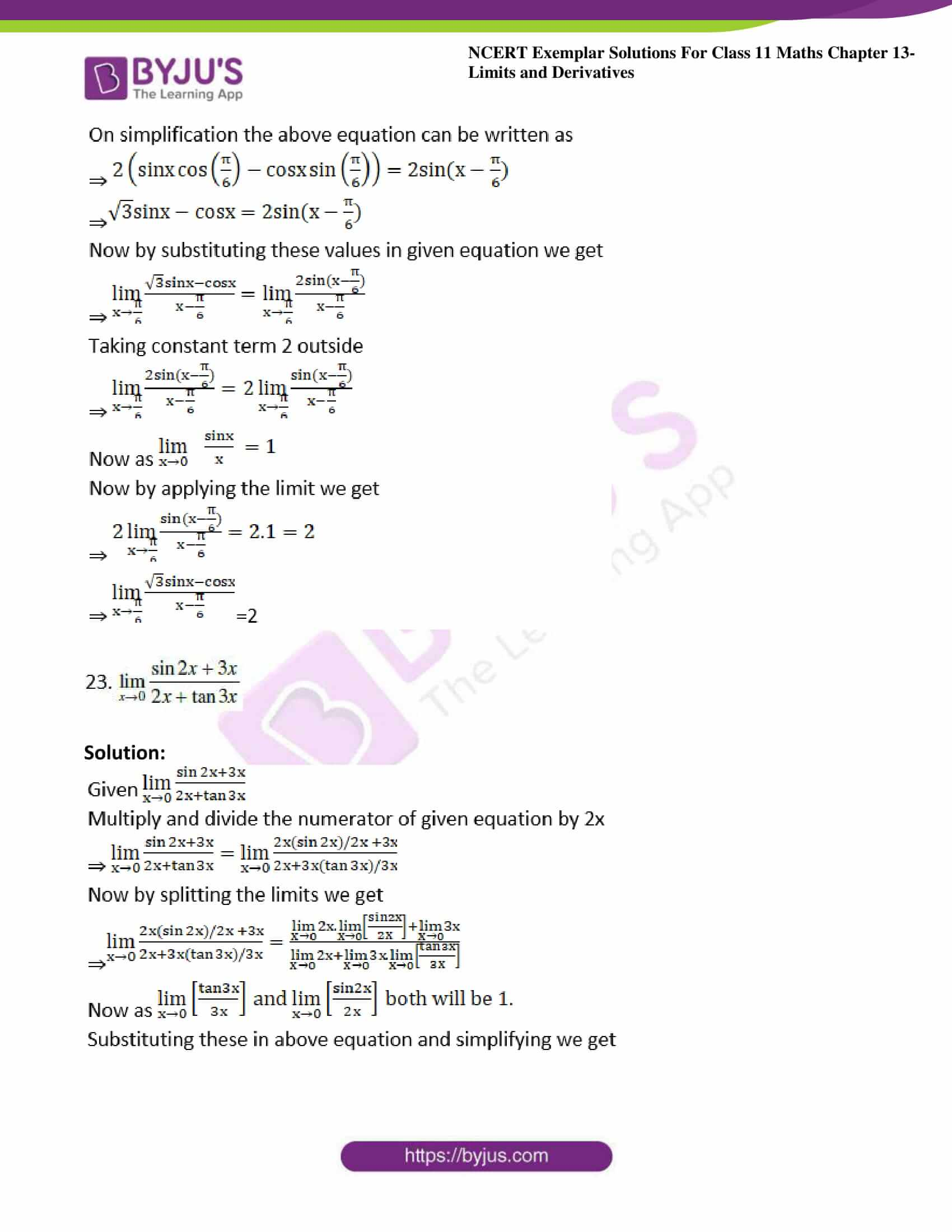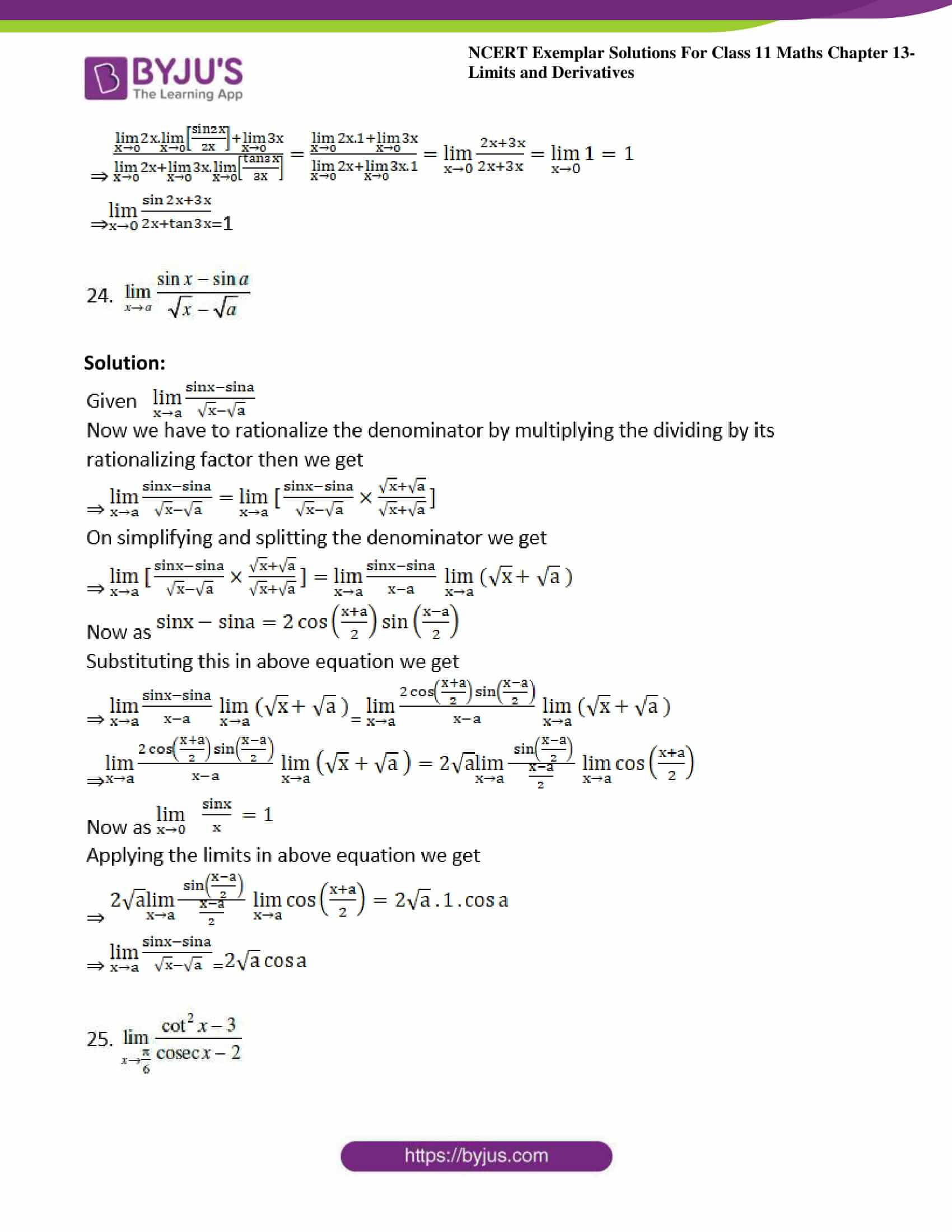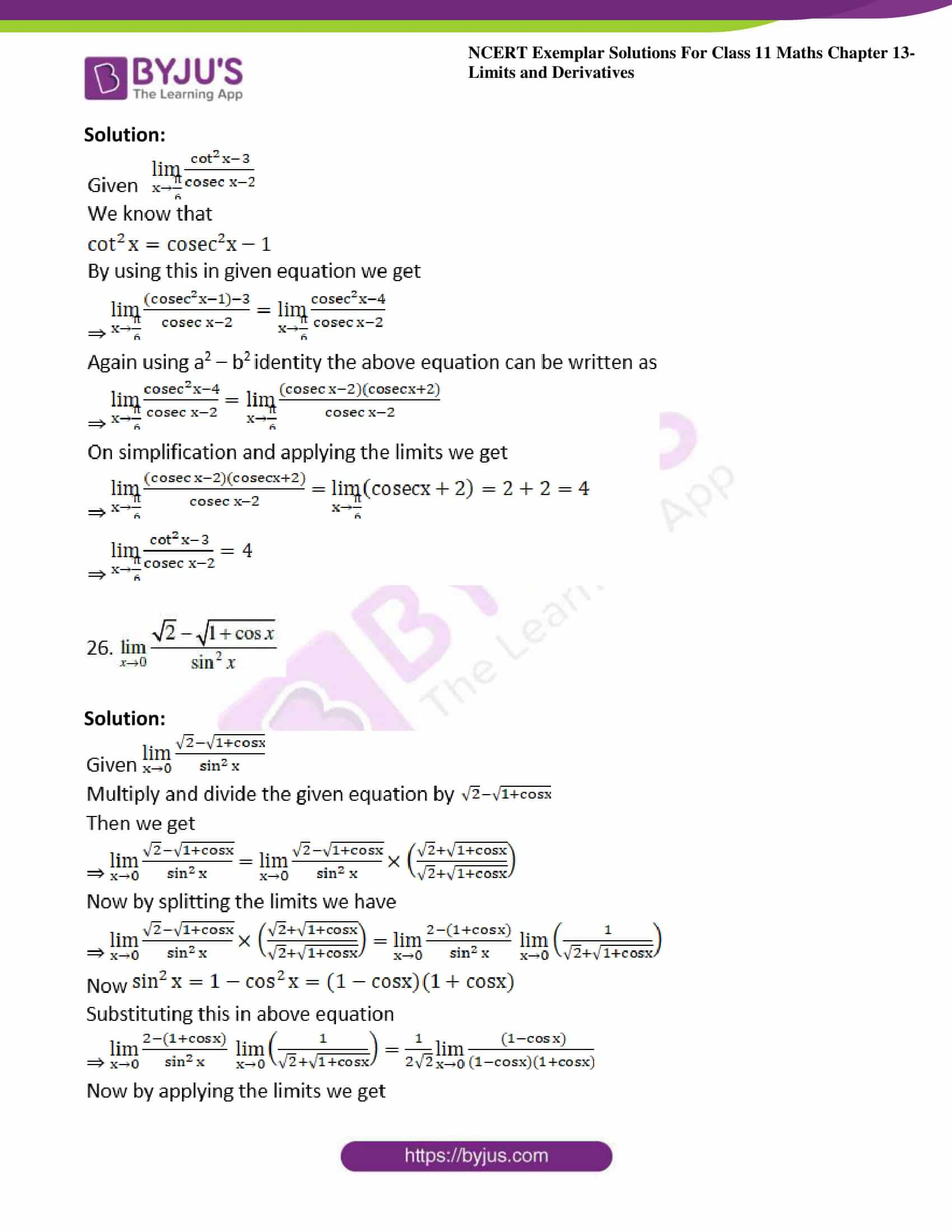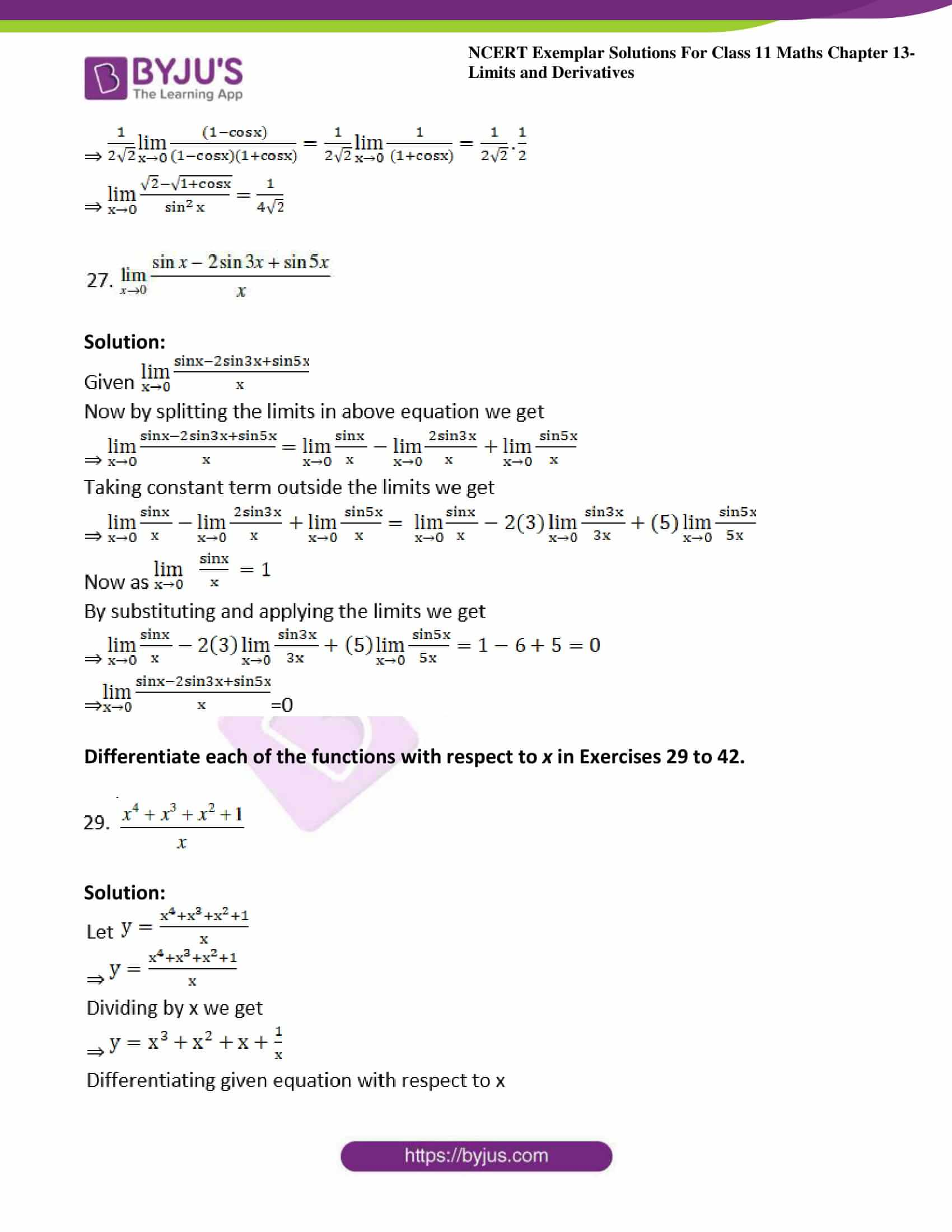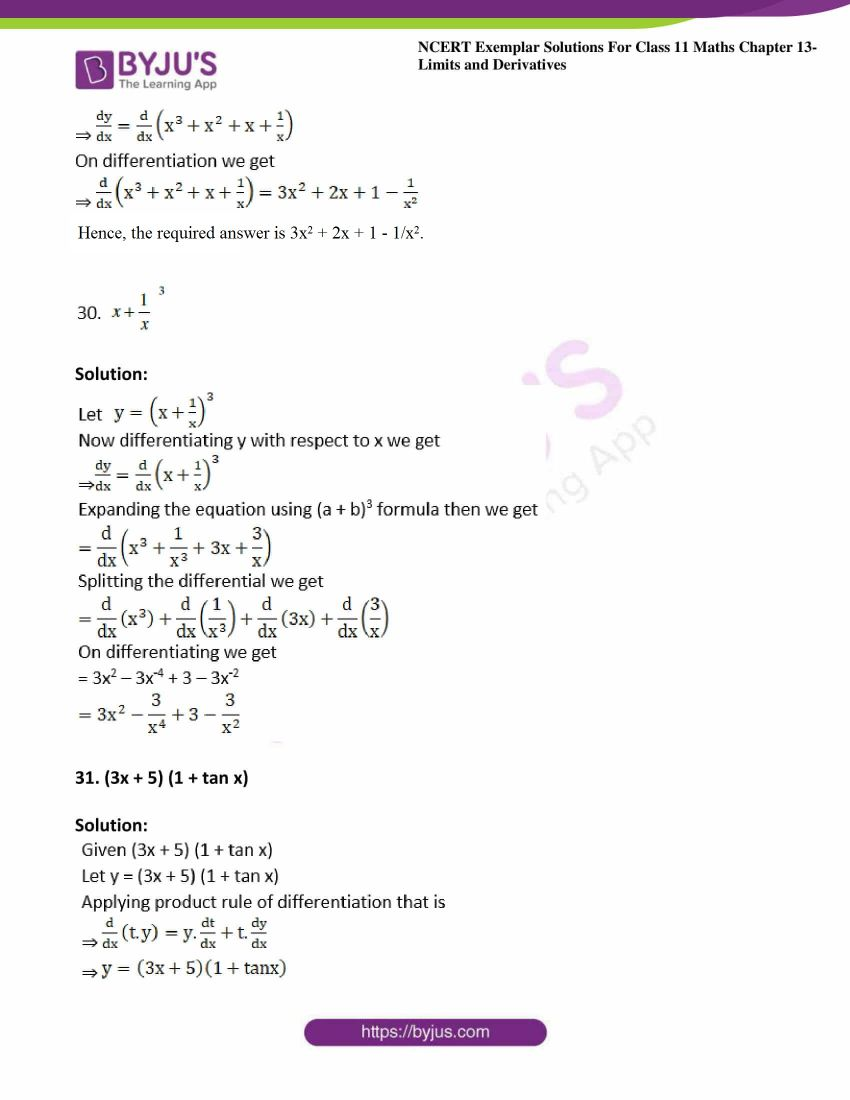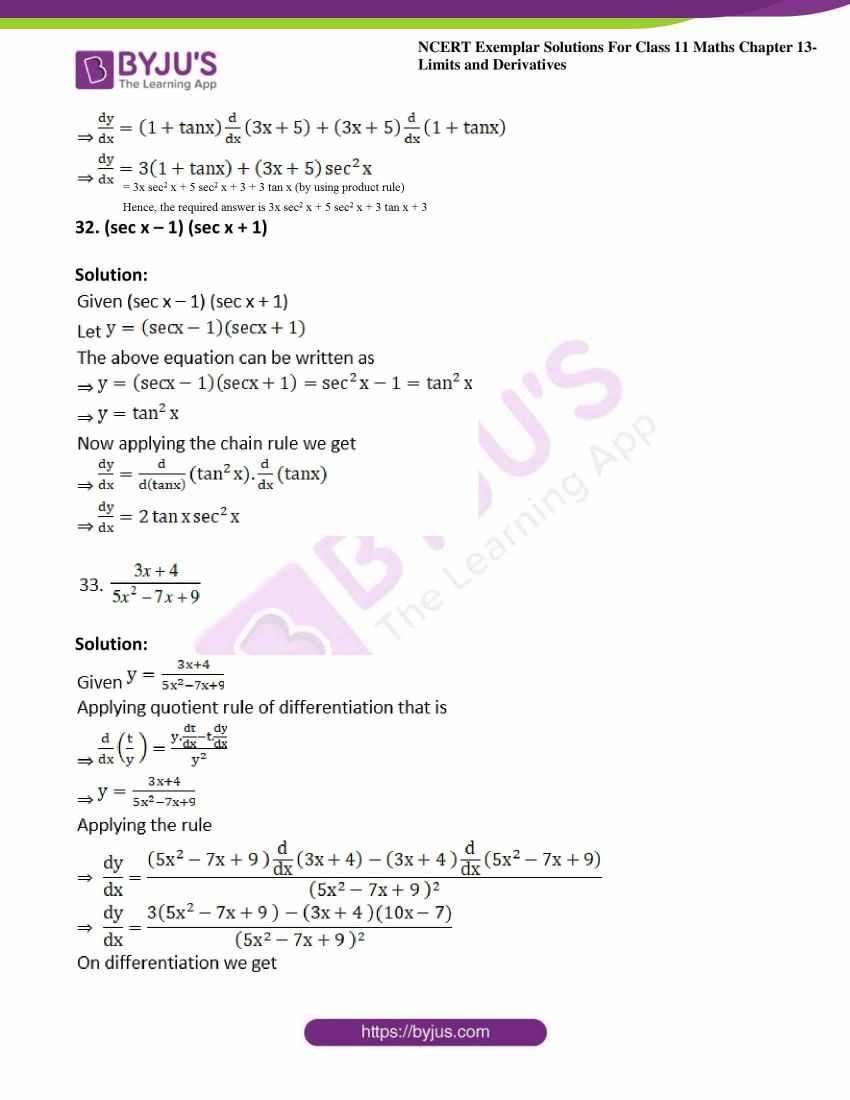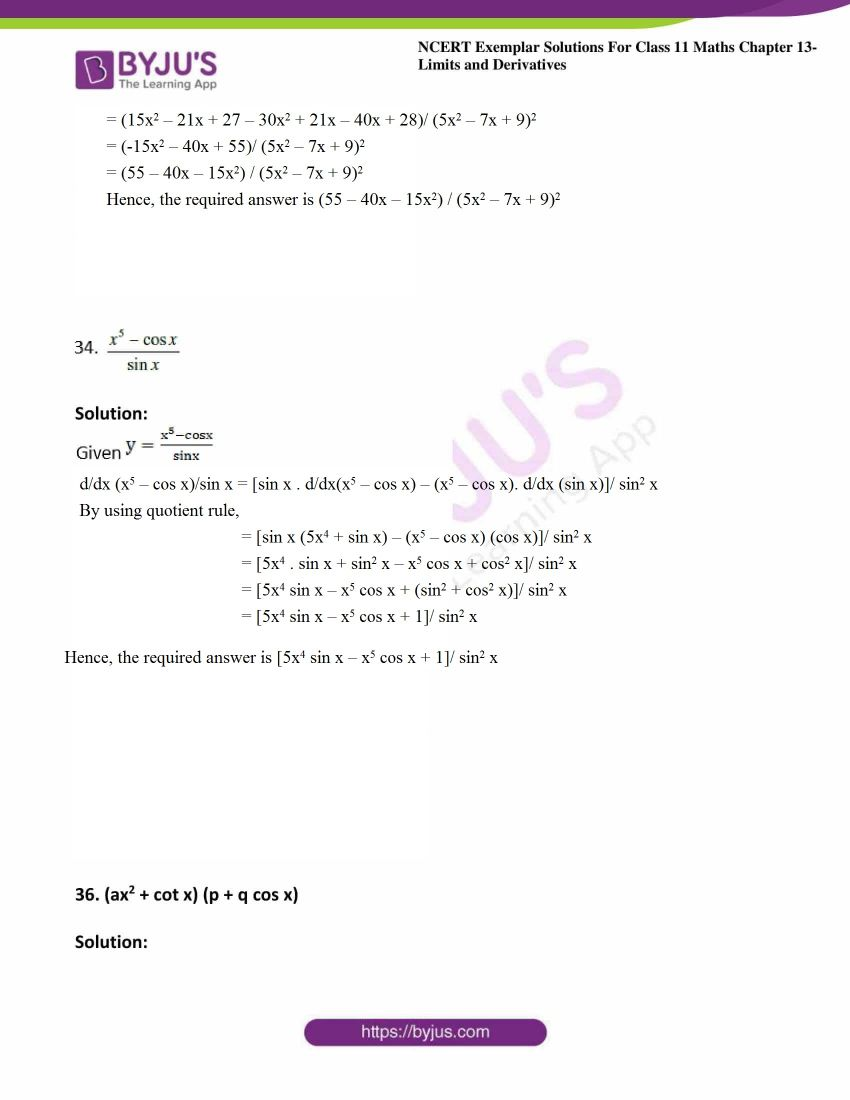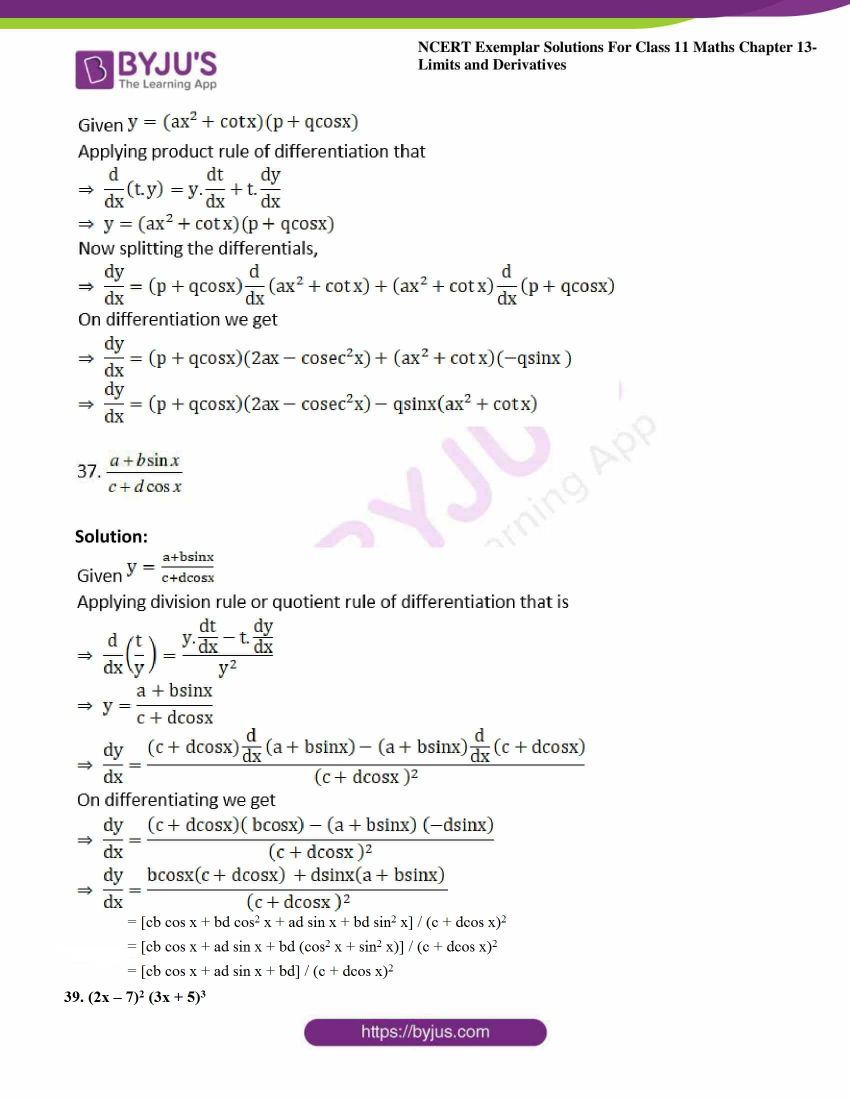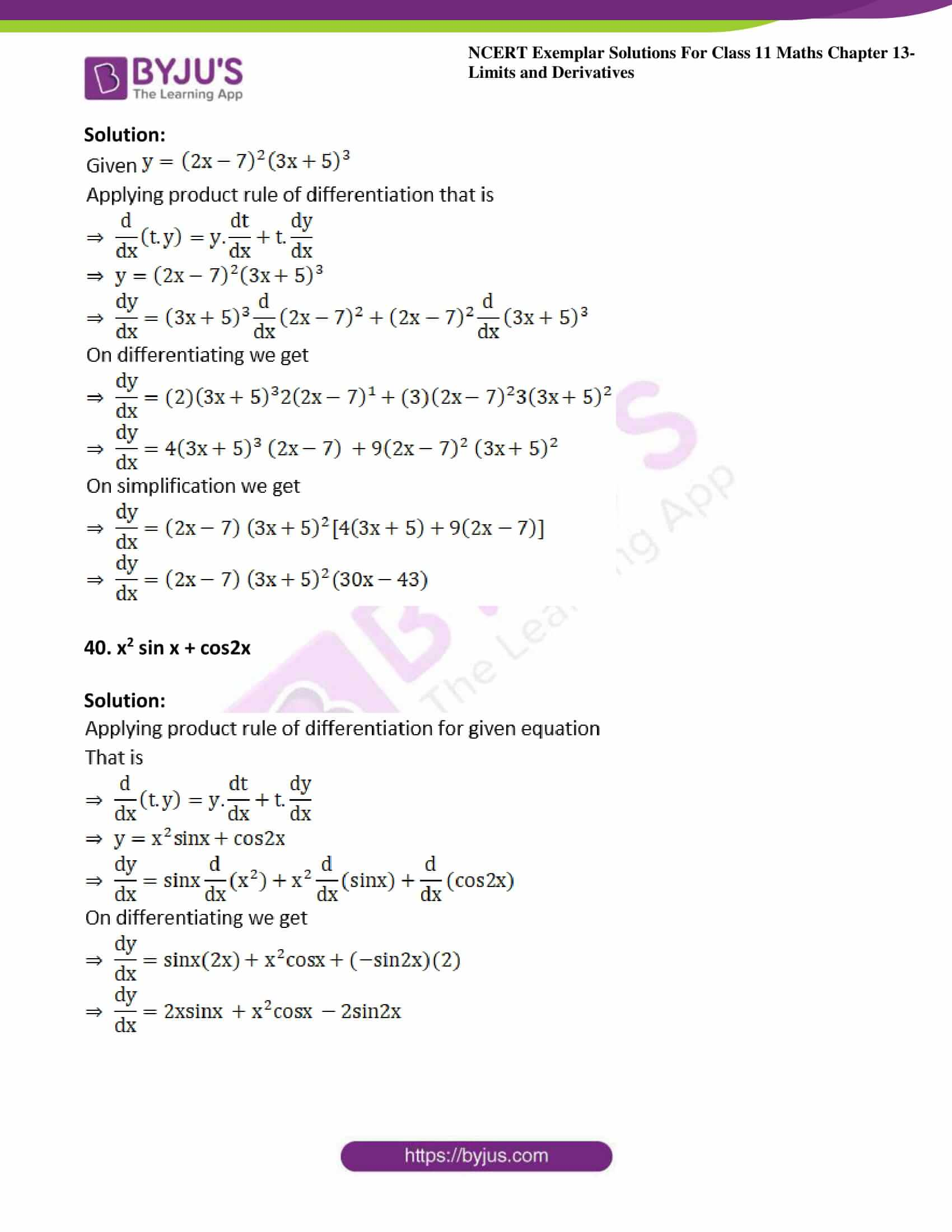### Access Answers to NCERT Exemplar Solutions for Class 11 Maths Chapter 13 – Limits and Derivatives

Exercise Page No: 239

Evaluate: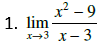Solution: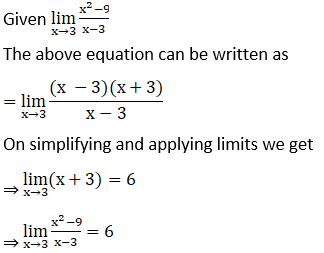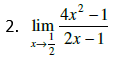Solution: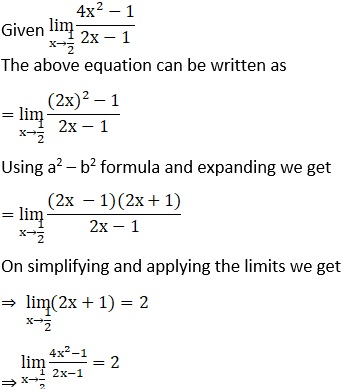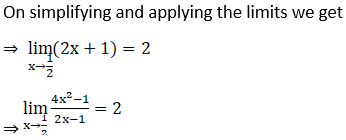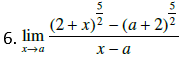Solution: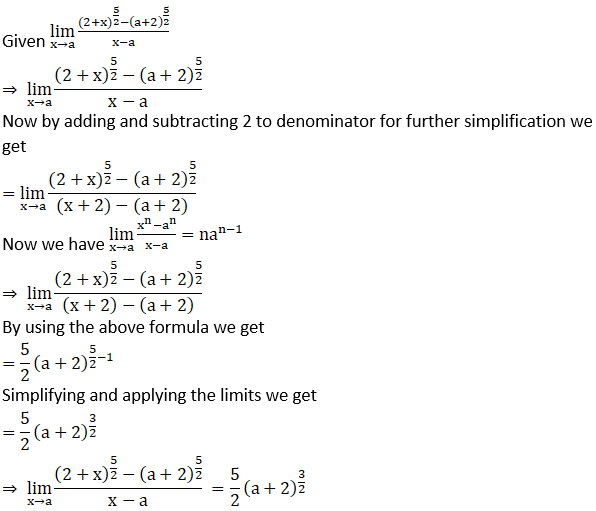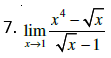Solution: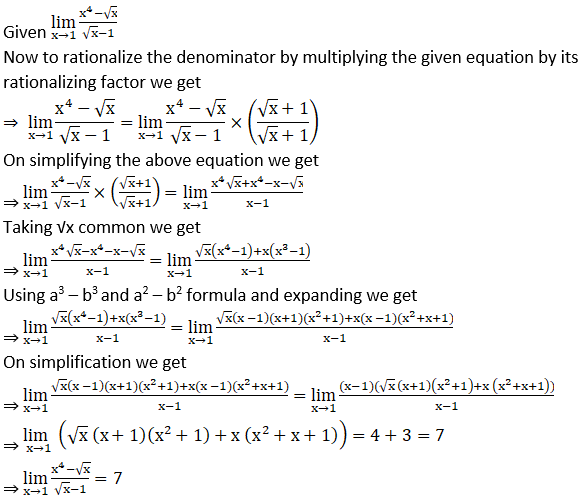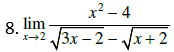Solution: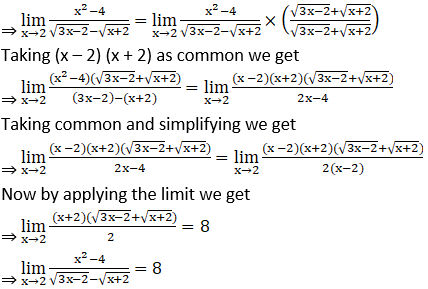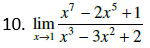Solution: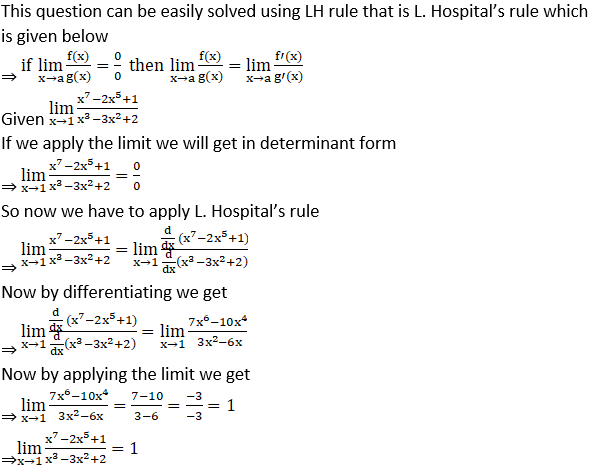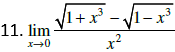Solution: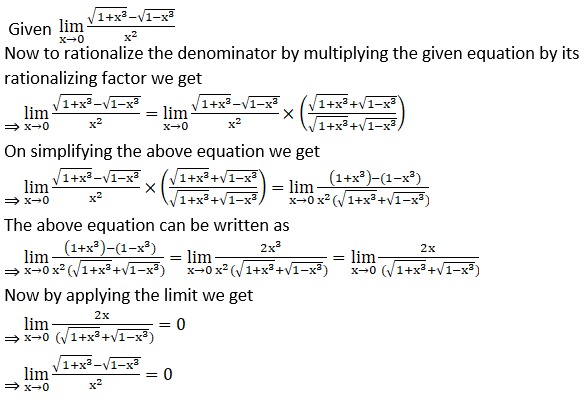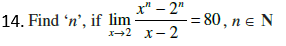Solution: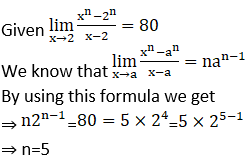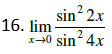Solution: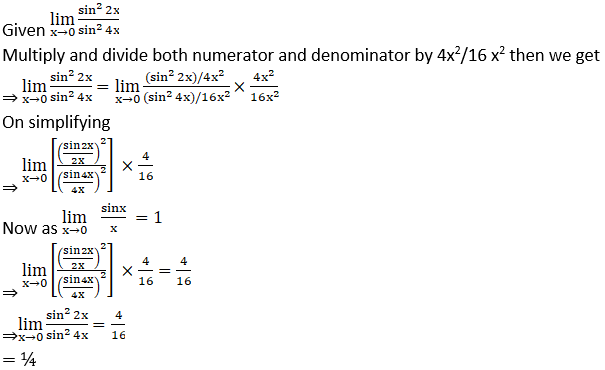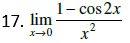Solution: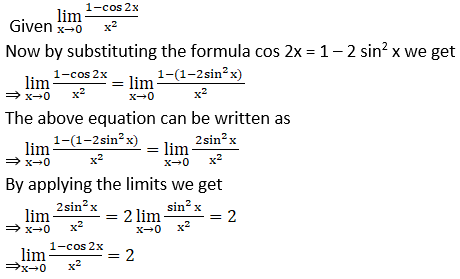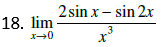Solution: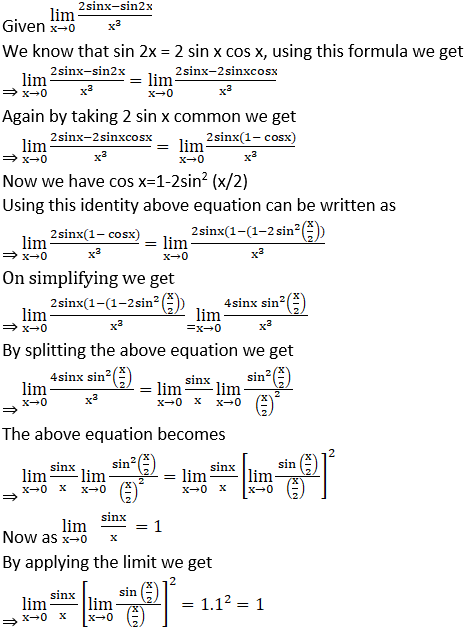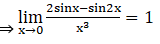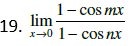Solution: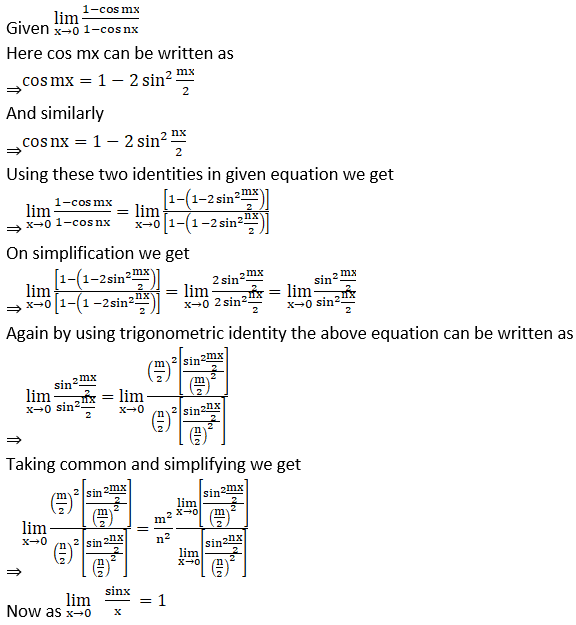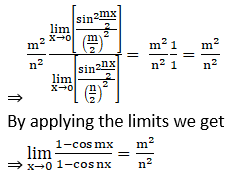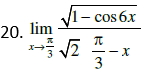Solution: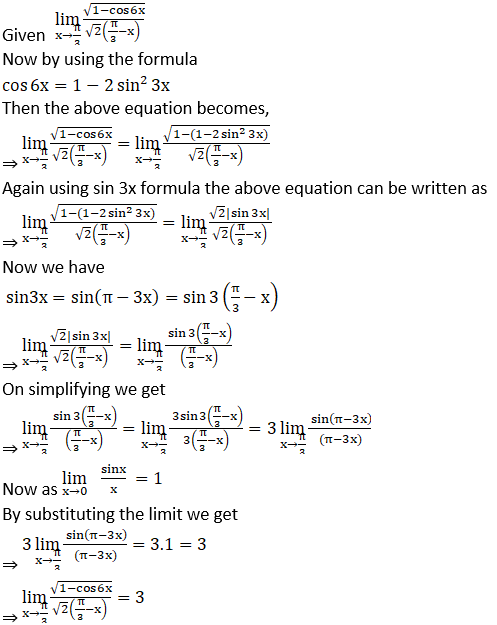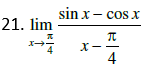Solution: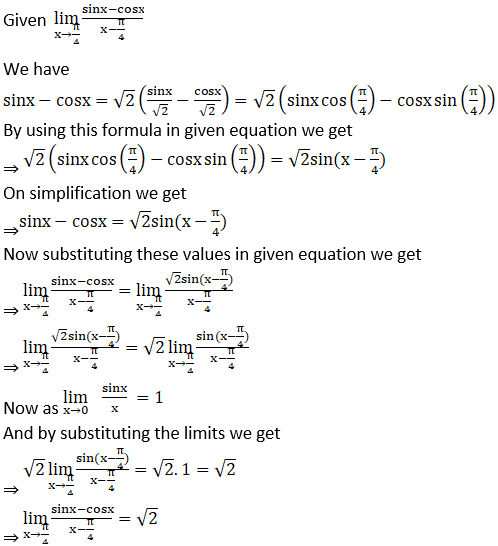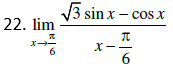Solution: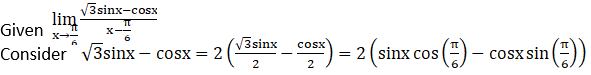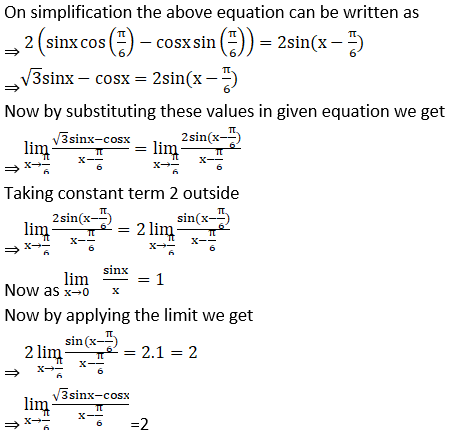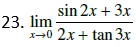Solution: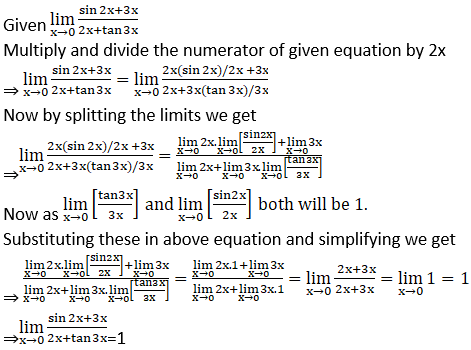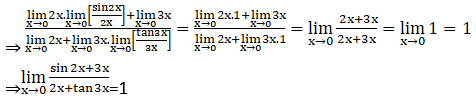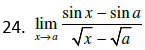Solution: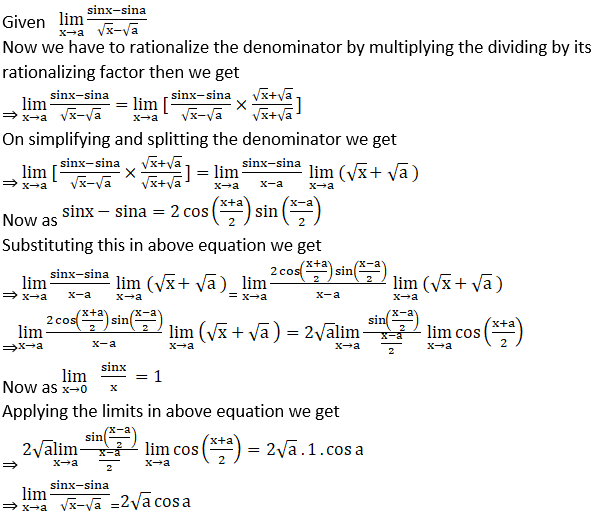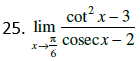Solution: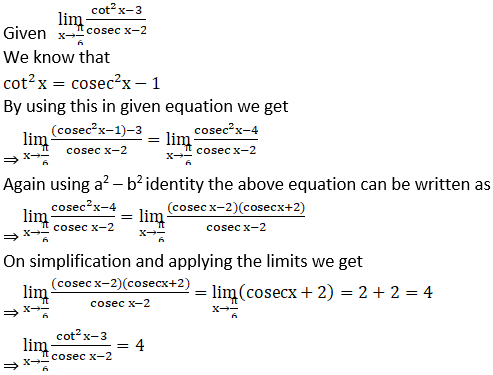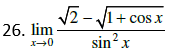Solution: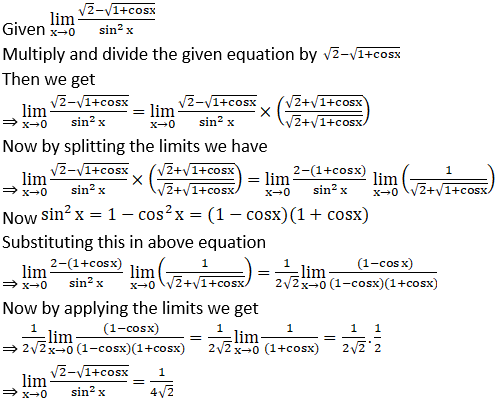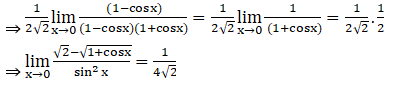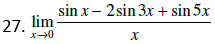Solution: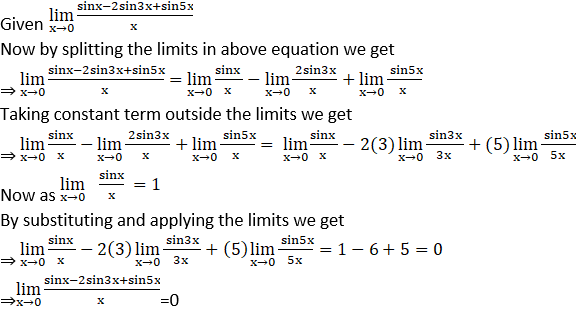Differentiate each of the functions with respect to x in Exercises 29 to 42.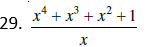Solution: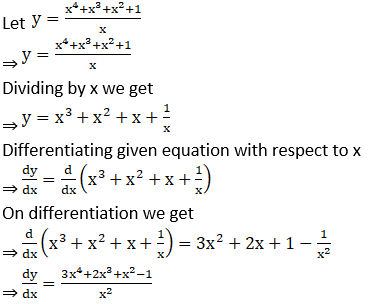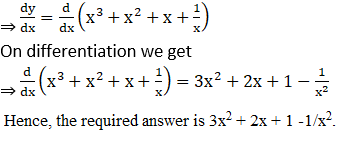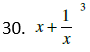Solution: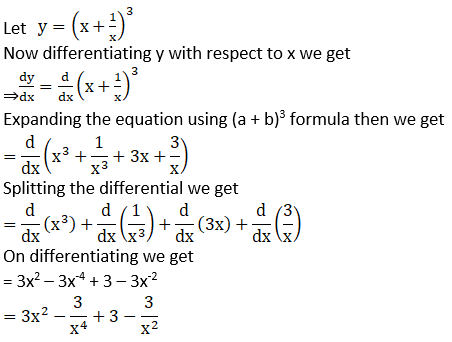31. (3x + 5) (1 + tan x)

Solution: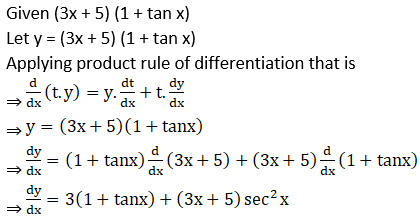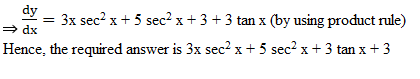32. (sec x – 1) (sec x + 1)

Solution: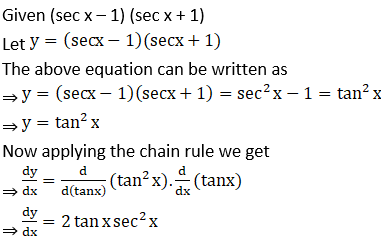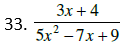Solution: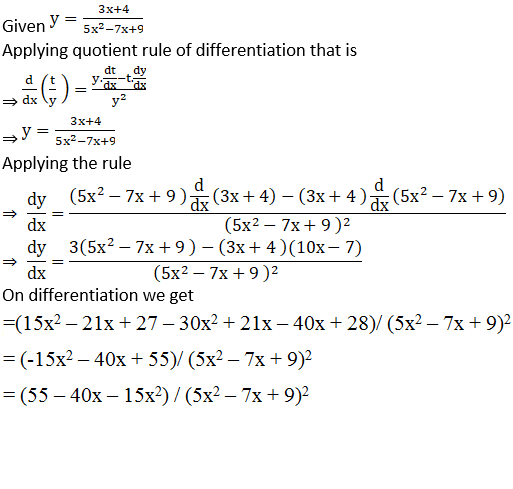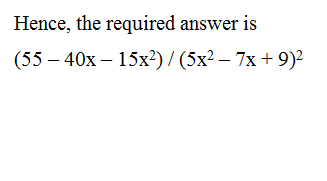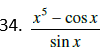Solution: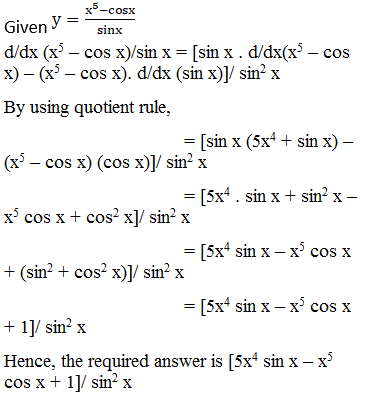36. (ax2 + cot x) (p + q cos x)

Solution: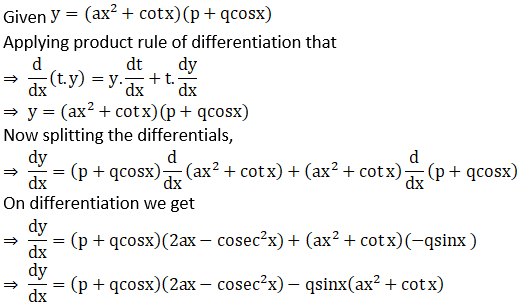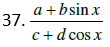Solution: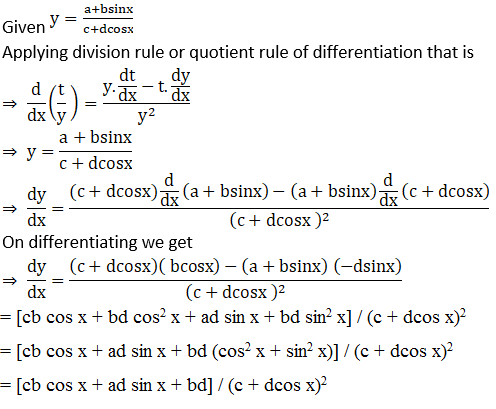39. (2x – 7)2 (3x + 5)3

Solution: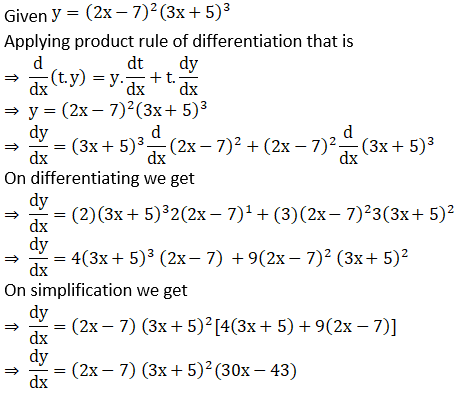40. x2 sin x + cos2x

Solution: